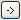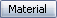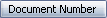Input Formula Functions

You can specify string and arithmetic functions to be used to configure the values returned by the input formula.

To access the functions:

Clicknext to Input Formula.

Arithmetic Functions

Function, Syntax, and Description Arguments and Examples

Returns the sum of the two specified numbers.

<term1> - First term

<term2> - Second term

Example: Add(2,3) - Returns 5
Subtract Numbers

Sub(<term1>,<term2>)

Returns the difference between the two specified numbers.

<term1> - First term

<term2> - Second term (amount to be subtracted)

Example: Sub(3,2) - Returns 1
Multiply Numbers

Multiply(<term1>,<term2>)

Returns the result of multiplying the two specified numbers.

<term1> - First term

<term2> - Second term (amount to multiply by)

Example: Multiply((3,2) - Returns 6
Divide Numbers

Divide(<term1>,<term2>)

Returns the result of dividing a number by a second number.

<term1> - First term

<term2> - Second term (amount to divide by)

Example: Divide(6,2) - Returns 3

String Functions

Function, Syntax, and Description Arguments and Examples
Left Substring

Left(<string>,<number>)

Returns the leftmost specified number of characters from a specified string.

<string> - The character string

<number> - The number of characters to be returned

Example: Left(ProjectAA,7) - Returns "Project"
Right Substring

Right(<string>,<number>)

Returns the rightmost specified number of characters from a specified string.

<string> - The character string

<number> - The number of characters to be returned

Example: Right(ProjectAA,2) - Returns "AA"
Middle Substring

Mid(<string>,<startpos>,<number>)

Returns a substring from a specified string (starting at a specified position).

<string> - The character string

<startpos> - The number of the starting position. The first character is position 0.

<number> - The number of characters to be returned

Example: Mid(ProjectAA,2,2) - Returns "oj"
String X from List

$Token(<string>|<string>|...,<index>,|) Returns the element number X from an ordered list where the elements are delimited with a certain character. This feature is useful in combination with controls connected to alias lists. <string> - List of strings to choose from. <index> - Index of string to use. This could be a variable linked to an alias list. | - The character used to separate the <string> values. Example:$Token(Metal|Plastic|Metal,,|)

Where the Material variable is connected to an alias list with the following values:
Displayed Value Alias
Steel 1
Polyester 2
Gold 3
The formula string becomes Metal if the user selects Steel or Gold as the material. It becomes Plastic if the user selects Polyester.
String Length

Length(<string>)

Returns the length of a specified string.

<string>
Example: Length(ProjectAA) - Returns 9
Find Substring

Find(<string>,<substring>)

Searches a specified string for the first match of a character or substring. Returns the position where the character or substring is found.

The first position in the character string is 0.
<string> - The character string

<substring> - The character substring for which to search

Example: Find(abc,b) - Returns 1
Reversed Find Substring

Reverse(<string>)

Returns the reverse of a specified string.

<string> - The character string
Example: Reverse(stockholm) - Returns "mlohkcots"
Reverse Find

ReverseFind(<string>,<substring>)

Searches a specified string for the last match of a character. Returns the position where the last occurrence of the character or substring is found.

The first position in the character string is 0.
<string> - The character string

<substring> - The character substring for which to search

Example: ReverseFind(abcabc,b) - Returns 4
Today's Date

$Time() Returns the current date in the format selected when the vault was created. Example:=$Time() - Returns 488a=2010-08-13